Open in App
Not now

# How to Enter Large Numbers/Credit Card Numbers into Microsoft Excel?

• Last Updated : 28 Jul, 2021

By default, in the cells of an Excel sheet, we canter 11 digits maximum. Once we enter a 12-digit number Excel changes it in terms of exponent and power notation.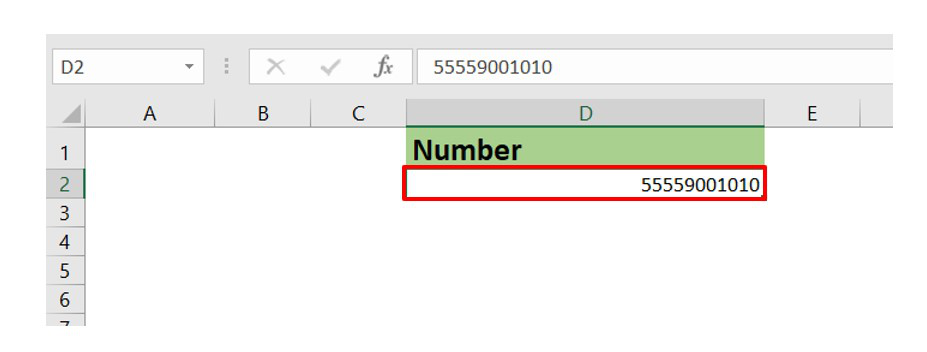11 digit number

Now, if we enter a 12 digit number let’s see what happens.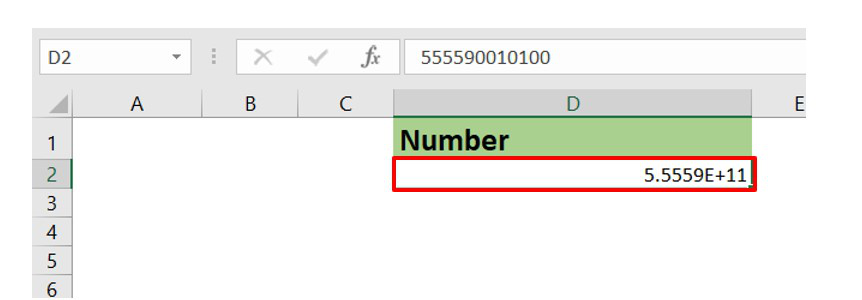We can observe that the representation of the 12-digit number becomes in terms of the power of 10 by converting to a decimal form. This is not a good representation since we can’t get the accurate precision of the number from the above representation.

In real life many times we deal with numbers having more than 11 digits. A great example is our Credit Card number which consists of 16 digits. So, we need an alternate way in Excel to store our Credit Card number with all the digits visible instead of decimal representation.

In this article, we are going to see how to enter large numbers in Excel using a credit card number having 16 digits as an example.

Example: Consider the dataset having the name, credit card number, expiry date of a geek.

Insert the data in the worksheet as shown below :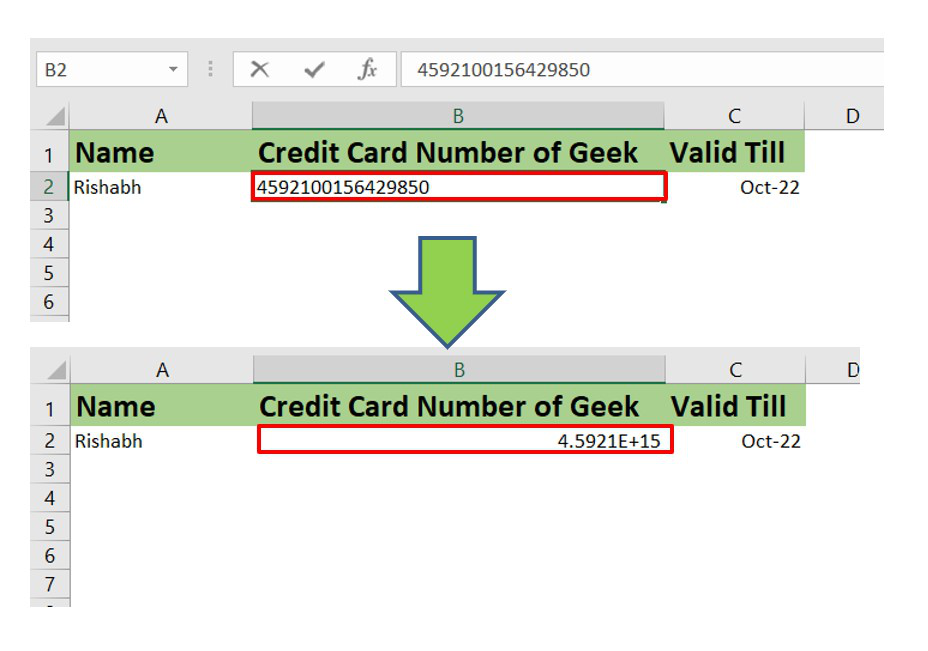We can observe as we enter the 16-digit number in the worksheet Excel automatically converts it into a decimal form.

So, to avoid it an alternate technique is there. The steps are :

• Right-click on the cell where you want to add a large number.
• A menu appears. Click on the Format Cells option.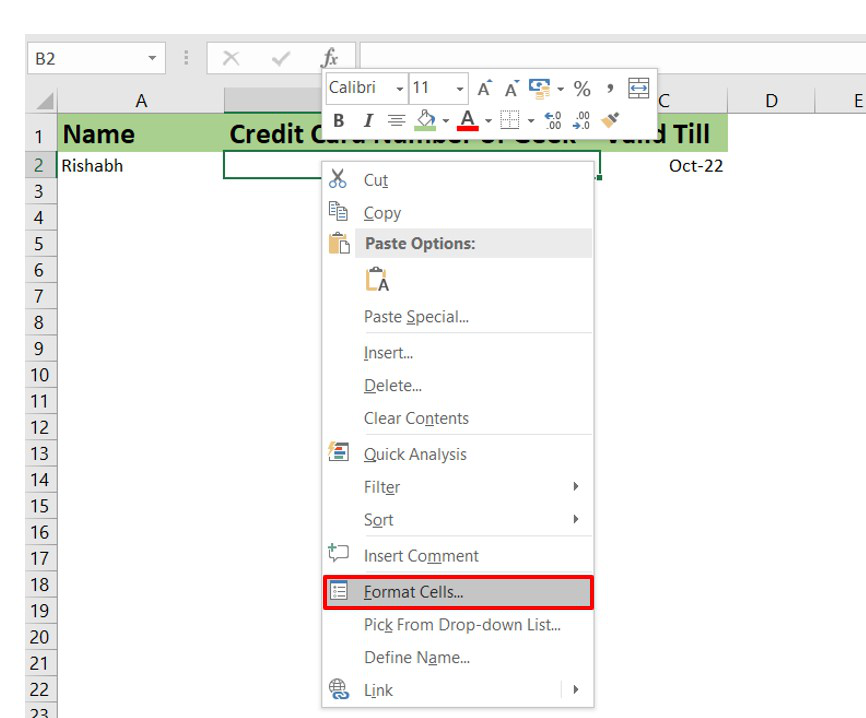• The Format Cells dialog box opens. In this click on the Number tab.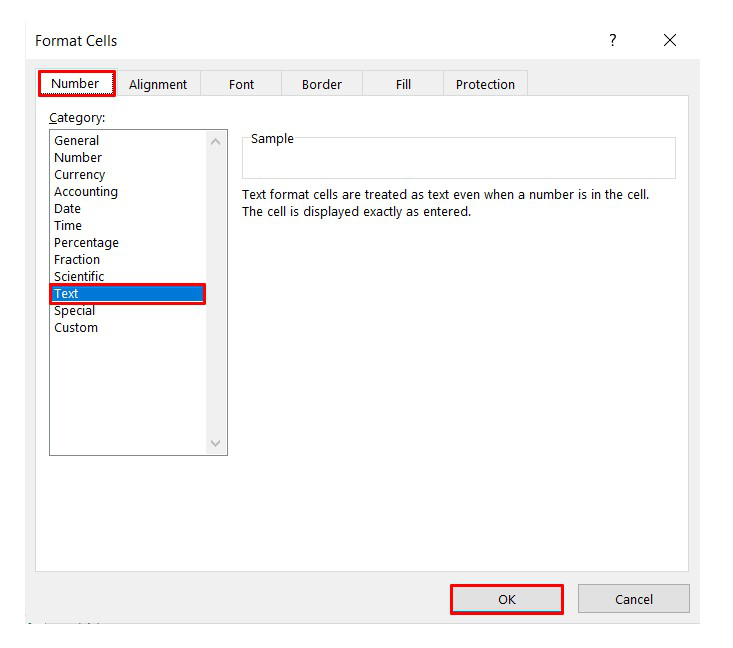• Now in the Category options select the Text option. This will format the cell from a number to a text. And we know a single Excel cell can read up to 32,767 characters in maximum. This will now prohibit the conversion of the credit card number into decimal form. This formatting will now consider each digit as a character.
• Click OK.
• Now re-enter the credit card number again and this time we will observe that we get the exact number and every digit is visible (with 100% precision) in the 16-digit number.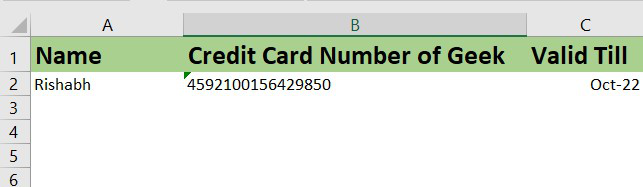In this way you can store a number in Excel containing more than 11 digits. It is a prediction that in upcoming updated versions of Excel, Microsoft will increase the limit up to 16 digits so that a credit card number can be stored easily without any formatting.

My Personal Notes arrow_drop_up